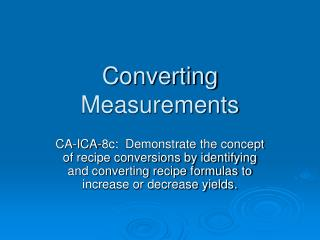DownloadDownload PresentationConverting Measurements

# Converting Measurements

Download Presentation## Converting Measurements

- - - - - - - - - - - - - - - - - - - - - - - - - - - E N D - - - - - - - - - - - - - - - - - - - - - - - - - - -
##### Presentation Transcript

1. Converting Measurements CA-ICA-8c: Demonstrate the concept of recipe conversions by identifying and converting recipe formulas to increase or decrease yields.

2. What is Converting? • When the yield of a recipe is not the same as the amount of product needed • Ex. A recipe produces 96 portions, but a chef needs 250 portions for a party.

3. Cost Per Portion • The conversion of a recipe will impact the cost of the recipe • The cost per portion however remains the same. • Ex. If a recipe for 24 costs \$5.25 per portion, the increasing the recipe for 36 would not change the price per portion.

4. Scaling • The process of calculating new amounts for each ingredient in a recipe when the total amount of food the recipe makes is changed

5. Scaling based on Yield • The most straightforward example of scaling a recipe is doubling the yield • Simply multiply all ingredients by 2 • 2 cups of milk becomes 4 cups of milk • ½ cup flour becomes 1 cup of flour

6. Scaling based on Yield • Becomes more difficult when the yield is changed by a factor that is not doubling or halving. • A recipe that normally yields 3 gallons scaled to yield 10 gallons • A recipe that makes 12 6-ounce portions may need to be scaled to make 30 9-ounce portions

7. Scaling Factor • The number that each ingredient amount in a recipe is multiplied by when the recipe yield is changed. SF = Desired Yield / Original Yield

8. Practice Find the Scaling Factor in each of the following. • A recipe makes 24 cookies. You want to change the recipe yield to 84 cookies. • A soup recipe makes 8 gallons of soup. You only need 3 gallons of soup. • A potato salad recipe makes 4 pounds of potato salad, but you need 80 pounds.

9. Check your Answers • SF = 84/24 • Scaling Factor = 3.5 • SF = 3 gal/8 gal • Scaling Factor = 0.375 • SF = 80 lb / 4 lb • Scaling Factor = 20

10. Scaling based on Portion Size A fish recipe makes 12 8-oz portions and it needs to be scaled to make 30 10-oz portions…. • The yields must first be converted to ounces Original Yield = 12 x 8-oz. = 96 oz. Desired Yield = 30 x 10-oz = 300 oz. • Then the original scaling formula can be applied. SF = 300 oz / 96 oz => SF = 3.125

11. Scaling based on Availability • A recipe may be scaled based on the availability of one ingredient • Ex. A recipe may require 20 pounds of ground beef but only 15 pounds of ground beef are available. • A foodservice worker needs to know how to adjust all of the other ingredients to account for the available amount of the key ingredient.

12. Scaling based on Product Availability SF = Available Amount / Original Amount • Beef stew recipe calls for 15 lbs of beef but needs to be made with 12 lbs of beef: SF = 12 lbs / 15 lbs => SF = 0.8

13. Multiplying Scaling Factors • Regardless of the method used for calculating the SF, the process for scaling the recipe is ALWAYS the same. • Once the SF is known, every ingredient amount is multiplied by the SF. New Amount (NA) = Original Amount x SF

14. Practice • 2 cups flour SF=2.5 • ½ c. water SF=.125 • 1 ½ T vanilla SF=10

15. New Amounts • It may be necessary to convert some of the new amounts to different units of measure • Makes for more efficient measuring • Ex. A recipe that once called for 1 tbsp oil, now calls for 8 tbsp of oil. • Ugh…kind of impractical to measure 1 tbsp of oil 8 TIMES!!!

16. How to Adjust Units • Convert to new unit 8 tbsp/1 x 1 fl oz/2 tbsp = 4 fl oz • Fl oz can then be converted to cups 4 fl oz/1 x 1 c/8 fl oz = ½ cup

17. Additional Concerns • Adjusting Measurements • Some measurements will not easily adjust such as 3.7 cups. Measurement will need to be adjusted to 3 ¾ cups (3.75 cups). • Sizing Cooking Equipment • Equipment must accommodate the adjusted amount of food…a smaller pan or larger pan. • Adjust Cooking Time • Adjust Cooking Temperature • Adjust Mixing Time